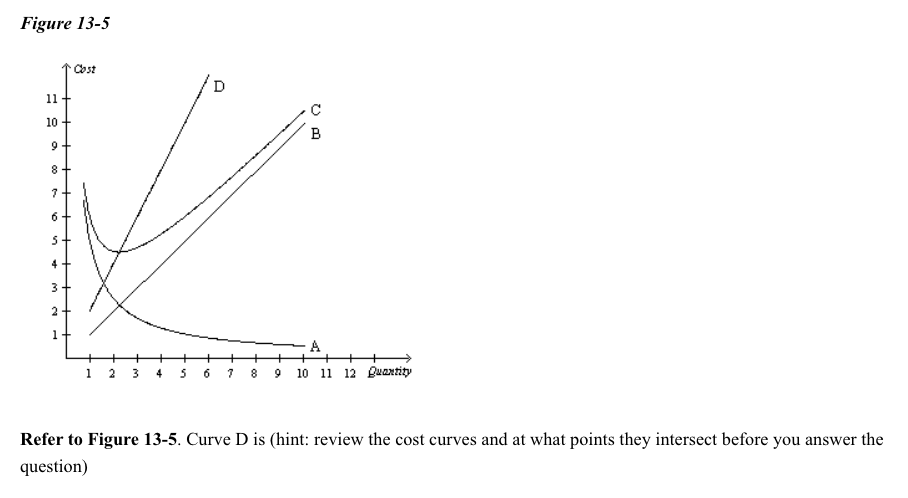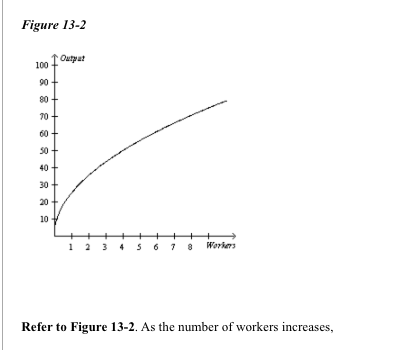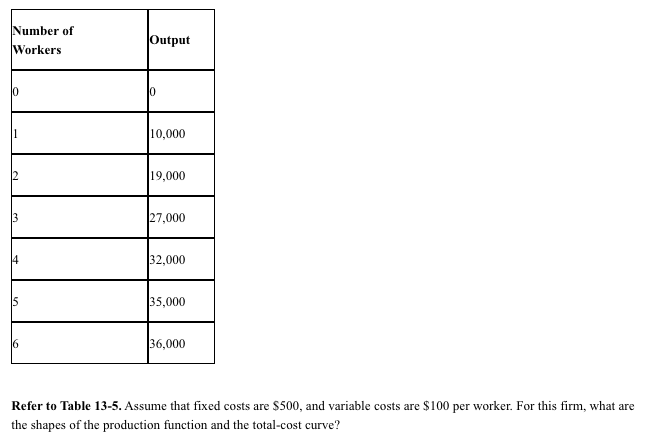# Question 1) A) Average Variable cost. B) Marginal Cost C) Average Fixed Cost D) Average Total Cost 2) If marginal product is 4 units and average product is 8 units, the next worker will Figure 13-5 1 Cost 1 2 3 4 5 6 7 8 9 10 11 12 Quantity Refer to Figure 13-5. Curve D is (hint: review the cost curves and at what points they intersect before you answer the question) Figure 13-2 Outpat 1 2 3 4 5 6 7 8 Workers Refer to Figure 13-2. As the number of workers increases, Number of Workers Output 10,000 19,000 27,000 32,000 35,000 136,000 Refer to Table 13-5. Assume that fixed costs are \$500, and variable costs are \$100 per worker. For this firm, what are the shapes of the production function and the total-cost curve?1)A) Average Variable cost.

B) Marginal Cost

C) Average Fixed Cost

D) Average Total Cost

2) If marginal product is 4 units and average product is 8 units, the next worker will cause

A) Average product to decrease.

B) Marginal product to increase by 2 units and average product to decrease by 2 units.

C) Average product to increase by 4 units.

D) Marginal product to become negative.

E) Marginal product to increase.

3)A) Both a and b are correct.

B) Marginal product decreases.

C) Total output decreases.

D) Marginal product increases but at a decreasing rate.

4)A) Both the production function and total-cost curve are increasing at a decreasing rate.

B) The production function is increasing at an increasing rate, whereas the total-cost function is increasing at a decreasing rate.

C) Both the production function and total-cost curve are increasing at an increasing rate.

D) The production function is increasing at a decreasing rate, whereas the total-cost function is increasing at an increasing rate.

Transcribed Image Text: Figure 13-5 1 Cost 1 2 3 4 5 6 7 8 9 10 11 12 Quantity Refer to Figure 13-5. Curve D is (hint: review the cost curves and at what points they intersect before you answer the question) Figure 13-2 Outpat 1 2 3 4 5 6 7 8 Workers Refer to Figure 13-2. As the number of workers increases, Number of Workers Output 10,000 19,000 27,000 32,000 35,000 136,000 Refer to Table 13-5. Assume that fixed costs are \$500, and variable costs are \$100 per worker. For this firm, what are the shapes of the production function and the total-cost curve?
More
Transcribed Image Text: Figure 13-5 1 Cost 1 2 3 4 5 6 7 8 9 10 11 12 Quantity Refer to Figure 13-5. Curve D is (hint: review the cost curves and at what points they intersect before you answer the question) Figure 13-2 Outpat 1 2 3 4 5 6 7 8 Workers Refer to Figure 13-2. As the number of workers increases, Number of Workers Output 10,000 19,000 27,000 32,000 35,000 136,000 Refer to Table 13-5. Assume that fixed costs are \$500, and variable costs are \$100 per worker. For this firm, what are the shapes of the production function and the total-cost curve?